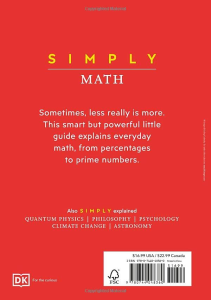# Simply Math By DK

PLDZ-36 In Stock
\$ 0.99 USD
Description

Combining bold, elegant graphics with easy-to-understand text, Simply Math is the perfect introduction to the subject for those who are short on time but hungry for knowledge.

Covering more than 80 key mathematical theories from prime numbers and matrices to logarithms and quadratic equations, each pared-back, single-page entry explains the concept more clearly than ever before.

Organized by major themes – number theory and systems; algebra, arithmetic, and calculus; probability and statistics; geometry and topology; logic, game theory, and computer science – entries demystify the groundbreaking ideas of famous mathematicians such as Pythagoras, Pierre de Fermat, Fibonacci, and Henri Poincaré, explaining the essentials of each key mathematical theory.

Whether you are studying math in high school or college, or simply want a jargon-free overview of the subject, this indispensable guide is packed with everything you need to understand the basics quickly and easily.

Recent Reviews Write a Review
0 0 0 0 reviews